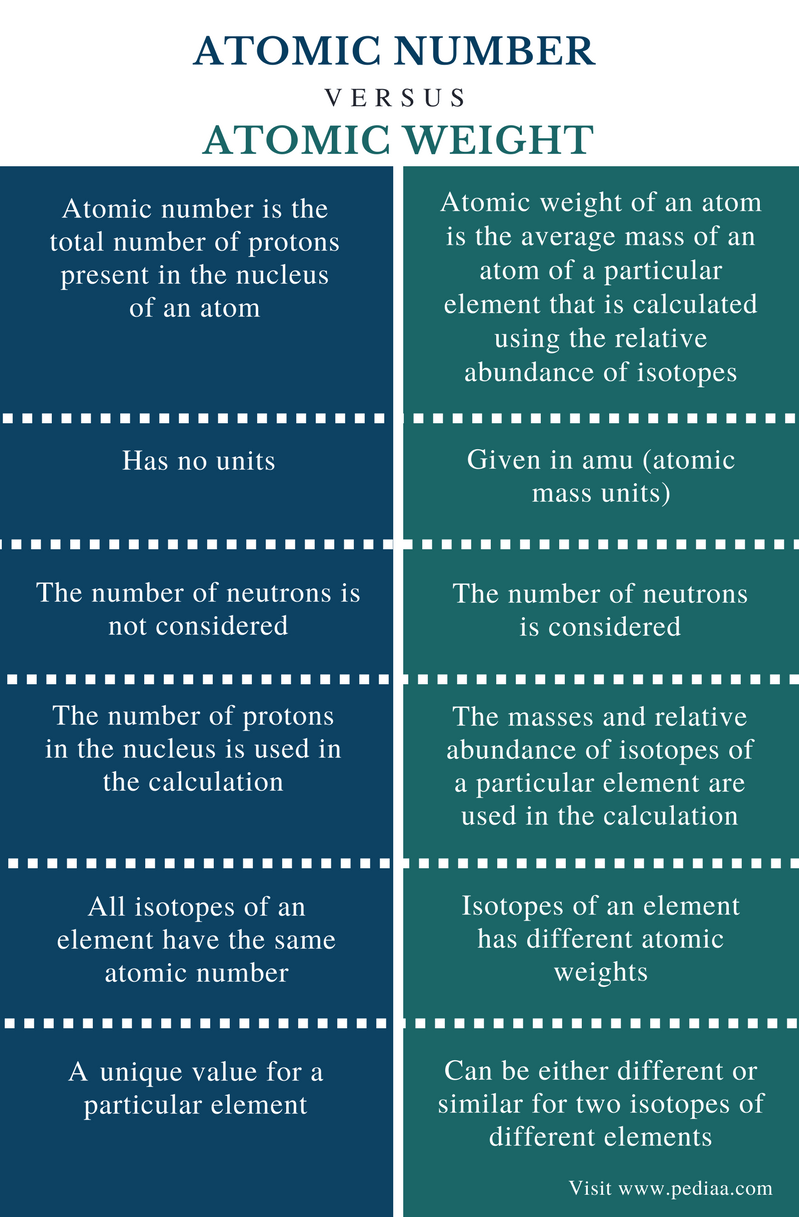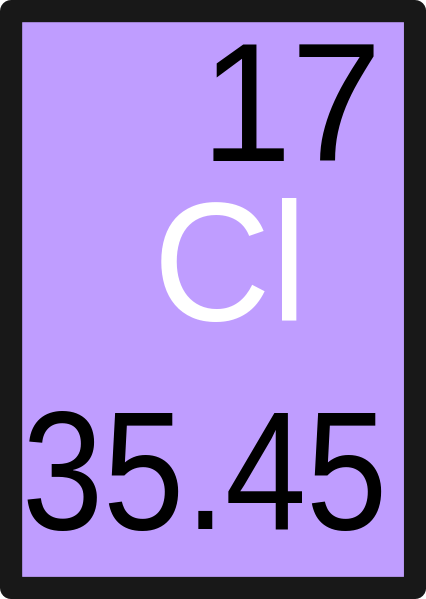# Difference Between Atomic Number and Atomic Weight

## Main Difference – Atomic Number vs Atomic Weight

An atom is the fundamental unit that all matter is made out of. Scientific discoveries revealed that an atom can be further divided into subatomic particles: electrons, protons, and neutrons. It was also discovered that an atom has a complex structure with a central core, named nucleus and electrons moving around this nucleus. The nucleus contains protons and neutrons. Atomic number and atomic weight are two chemical terms that are used to explain the number of subatomic particles present in an atom and its mass. The main difference between atomic number and atomic weight is that atomic number gives the number of protons present in the nucleus whereas atomic weight gives the mass of an atom.

### Key Areas Covered

1. What is Atomic Number
– Definition, Explanation
2. What is Atomic Weight
– Definition, Explanation
3. What is the Difference Between Atomic Number and Atomic Weight
– Comparison of Key Differences

Key Terms: Atom, Atomic Number, Atomic Weight, Electrons, Neutrons, Nucleus, Protons## What is Atomic Number

Atomic number is the total number of protons present in the nucleus of an atom. The protons are in the nucleus of an atom. The number of protons in an atom is a unique property for a chemical element. Therefore, we can identify a given chemical element by finding the number of protons present in its atom.  Each and every atom has at least one proton. For example, a hydrogen atom has only one proton.

Atomic number is a unit-less measure since it gives only the number of protons. Isotopes are atoms that have the same number of protons but different numbers of neutrons. Since these isotopes have the same number of protons, they belong to the same chemical element. Therefore, when we are talking about isotopes, we are talking about the different forms of the same element that has the same atomic number.Figure 1: The atomic number of Helium is 2. There are two protons in the helium nucleus.

For a neutral atom, the number of protons in the nucleus is equal to the number of electrons that are in movement around the nucleus. Hence the atomic number of a certain element expresses the number of electrons present in a neutral atom of that element.

The atomic number of an element can be changed if that element is radioactive. This is because protons are converted into different subatomic particles or neutrons may be converted into protons when some elements undergo radioactivity. Either way, it changes the number of protons present in an atom. This changes into a different element (change in atomic number changes the element).

## What is Atomic Weight

Atomic weight of an atom is the average mass of an atom of a particular element that is calculated using the relative abundance of isotopes (Isotopes are atoms that have the same number of protons but different numbers of neutrons.). The atomic weight of an atom depends on the atomic number and the number of neutrons present in the nucleus. Since the mass of an electron is considerably small, it is negligible. Therefore, the atomic weight does not depend on the number of electrons.Figure 2: Atomic Weight of Chlorine

The unit atomic weight is calculated as one twelfth of the weight of a carbon-12 isotope in its ground state. This carbon-12 atom has 12 atomic mass units. Let us consider an example.

Atomic Weight of Chlorine (Cl):

Most abundant isotopes of chlorine are Cl-37, Cl-36 and Cl-35.

 Isotope Abundance (%) Mass (amu) Cl-35 76 34.96 Cl-37 24 36.96

Atomic weight of chlorine = (34.96 amu x 76%) + (36.96 amu x 24%)
= (26.57 + 8.87) amu
= 35.44 amu

The atomic weight of chlorine is about 35.44 amu. Likewise, we can calculate the atomic weight of any element considering the isotopes and their abundance.

## Difference Between Atomic Number and Atomic Weight

### Definition

Atomic Number: Atomic number is the total number of protons present in the nucleus of an atom.

Atomic Weight: Atomic weight of an atom is the average mass of an atom of a particular element that is calculated using the relative abundance of isotopes.

### Unit

Atomic Number: Atomic number has no units.

Atomic Weight: Atomic weight is given in amu (atomic mass units).

### Neutrons

Atomic Number:  The number of neutrons is not considered when calculating the atomic number.

Atomic Weight: The number of neutrons is considered when calculating the atomic weight.

### Calculation

Atomic Number: The number of protons in the nucleus is calculated to get the atomic number.

Atomic Weight: The masses and relative abundance of isotopes of a particular element are considered in calculating its atomic weight.

### Isotopes

Atomic Number: All isotopes of an element have the same atomic number.

Atomic Weight: Isotopes of an element has different atomic weights.

### Value

Atomic Number: The atomic number is a unique value for a particular element.

Atomic Weight: The atomic weight can be either different or similar for two isotopes of different elements.

### Conclusion

Atomic number and atomic weight are two important chemical terms. These are two fundamental facts about an atom. The main difference between atomic number and atomic weight is that atomic number gives the number of protons present in the nucleus whereas atomic weight gives the mass of an atom.

##### References:

1. Helmenstine, Anne Marie. “How To Calculate Atomic Weight.” ThoughtCo, Available here.
2. Helmenstine,  Anne Marie. “What Is Atomic Weight? Here’s What You Should Know.” ThoughtCo, Available here.
3. “Atomic number.” Dictionary.com, Available here.

##### Image Courtesy:

1. “Atomic number depiction” By Tom29739 – Own work (CC BY-SA 4.0) via Commons Wikimedia
2. “Chlorine” By me – Image:Chlorine.gif (CC BY-SA 2.5) via Commons Wikimedia